# Physical Quantities

Welcome to class!

In today’s class, we will be talking about physical quantities. Enjoy the class!

##Physical quantities are properties of a phenomenon that can be determined by calculation or measurement from a reference point using an instrument.

#### Types of quantities

Physical quantities are classified into two. Namely:

• ##### Scalar Quantities:

Scalar quantities are those quantities that have only magnitude/size. They do not have directions.

Examples include:

1. Distance
2. Time
3. Length
4. Temperature
5. Speed
6. Volume
7. (7) Density
8. (8) Area

Scalar quantities are added by ordinary algebraic methods.
Examples:
(1) V1 + V2 = 5 cm³ + 10 çm³ = 15 cm³
(2) L1 + L2 =  25 m + 46 m = 71 m

• ##### Vector Quantities:

Vector quantities are those quantities that have both magnitudes/sizes and directions/parts.

Examples include:

1. Force
2. Weight
3. Electric field
4. Gravitational field.
5. Displacement
6. Momentum
7. Velocity
8. Acceleration
###### Representation of Vector

A vector quantity is represented by a line with an arrowhead. The length of the line represents the size or magnitude of the vector quantity while the arrowhead represents the direction of travel of the as shown below:

Addition of vectors is also called equivalent vector or resultant vector or effective vector. To find the sum of the two vectors, the two vectors are added together putting into consideration the negative sign of the negative vector as shown below.

###### How to Find the Resultant Vector of Perpendicular vectors

The resultant vector of two vectors that travelled in a perpendicular direction one to another cannot be found by the mere addition of vectors. Rather, a vector triangle is used to find the resultant of the vectors. The length of the hypotenuse represents the magnitude/size of the resultant vector. Pythagoras formula is then used to calculate the resultant vector.

Thus,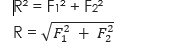##### The direction of the Resultant Vector

The direction of a resultant vector is the angle which resultant vector makes with the horizontal.

###### The formula for Calculating Direction of Resultant Vector

The formula for calculating the direction of a resultant vector is given below.##### Resultant Vector

A resultant vector is a single vector that will do the same amount of work, in the same direction that many vectors acting together would have done.

##### Methods of Finding Resultant Vector

There are two methods of finding the resultant vector of two or more vectors acting from a common point:

1. The Parallelogram method
2. The triangle method
###### The Parallelogram Method of Finding Resultant Vector

If two vectors act at an angle of to one another and act from a common point, the parallelogram method is used to find the resultant of the two vectors. In a parallelogram method, the two vectors are represented by the adjacent sides of the parallelogram while the diagonal represent the resultant vector.

Parallelogram Law of Vector

The parallelogram law of vector states that if two vectors act from a common point at an angle to each other, the vectors can be represented in magnitude and direction by the adjacent sides of the parallelogram. The diagonal of the parallelogram represents the resultant vector in magnitude and direction.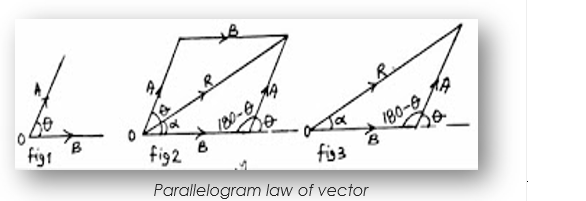From figure 3, let OA represent vector A and OB represent vector B while OR represent vector R  we will use cosine  thus:

R2 = OA2 + OB2 – 2(OA) x (OB) Cos (180 – )is the angle between the two vectors

###### WORKED EXAMPLE

(1) Calculate the resultant of two vectors of 4 units and 7 units acting at a point at an angle of 60° to each other. Calculate the direction of the vector.

SOLUTION
The question is as shown in the figure by your right.

Data given in the question:

F1 = AO = 4 units, F2 = OB = 7 units, = 60°, R = UnknownThe diagram is as shown by your right:

Formula:

R2 = OA2 + OB2 – 2(OA) x (OB) Cos (180 – )

Substituting: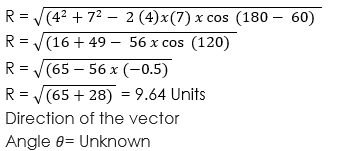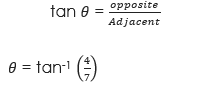##### Analytical Method of Finding Resultant Vector

In the analytical method of finding the resultant vector of two vectors, the data given in e question are used to draw or construct a parallelogram to scale. Then the diagonal of the parallelogram is measured to determine its magnitude.

If the parallelogram is drawn accurately to scale, the measured resultant will be approximate as the calculated value.

###### Triangle Law of Vector

Triangle law of vector states that if two vectors act from a common point, the two vectors can be represented in magnitude and direction by the two sides of a triangle while the third side represents in magnitude and direction.

###### Definition of Components of A Vector

Component of a vector in a given direction is the effective value of that vector in that direction. The horizontal component of a vector is the effective value of that vector in the horizontal direction while the vertical component of the vector is the effective value of the vector in the vertical direction.

###### WORKED EXAMPLES

(1) A force of 23 N acts at an angle of 60° to the horizontal. Calculate the components of the vector.

SOLUTION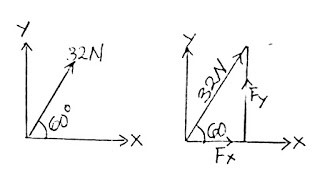Data given in the question:

Force F = 32N,

Angle= 60°

Vertical component Fy:

Fy = F x SinSubstitution:             Fy = 32 x Sin 60°

Fy = 32 x 0.8660

Fy = 27.712N

Horizontal Component Fx:

Fx = F x CosSubstitution:             Fx = 32 x Cos 60°

Fx = 32 x 0.5

Fx = 16N

(2) The vertical component of a force F is 12 N. If the angle which the force makes with the vertical is 30°, calculate the value of F and the horizontal component of the force.

SOLUTION
Force F:

Data given in the question:

F = Unknown,

Angle= 30°

Vertical component Fy = 12N.

Fy = F x Sin 30°

Substitution:         12 = F x Sin 30°

12 = F x 0.5

Make F the subject:

F = 12/0.5 = 24N

Horizontal component Fx:

Fx = F x Cos

Substitution:         Fx = 24 x Cos 30°

.                               Fx = 24 x 0.8660 = 20.78N

###### Resolution of Vectors

Resolution of vectors is a process of resolving each vector into its vertical direction and horizontal direction and then add all the vertical components/direction and all the horizontal components/direction to obtain a single vertical direction and single horizontal direction after which the resultant is found.

WORKED EXAMPLE

(1) The resultant vector of two vectors are 32 N. If the angle between the vectors is 45°, calculate Q if the other vector is 8N.

SOLUTION
Given parameters:R = 32N, F1 = OA = q N, F2 = OB = 8 N, angle= 45°

From figure 3,

Formula:

R2 = OA2 + OB2 – 2(OA) x (OB) Cos (180 –)

Substituting:

322 = q2 + 82 – 2(q) x (8) Cos (180 – 45 )

1024 = q2 + 64 – 16q Cos (135)

1024 = q2 + 64 – 16q (–0.7071)

1024 = q2 + 64 + 11.31q

q2 + 11.31q + 64 – 1024 = 0

q2 + 11.31q – 960 = 0

Since we derived at quadratic expression, to calculate q as follow:a = 1, b = 11.31 and c = – 960

Substitution: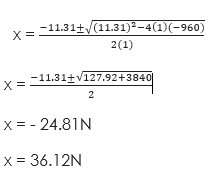We ignore the negative value and take the positive value of the force.
Therefore, the force q = 36.12N

In our next class, we will be talking about Equations of Motion.  We hope you enjoyed the class.

Should you have any further question, feel free to ask in the comment section below and trust us to respond as soon as possible.

How Can We Make ClassNotesNG Better - CLICK to Tell Us💃